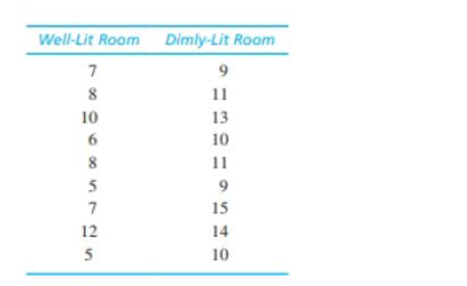Chapter 10, Problem 24PEssentials of Statistics for the B...

8th Edition
Frederick J Gravetter + 1 other
ISBN: 9781133956570

Solutions

Chapter
SectionEssentials of Statistics for the B...

8th Edition
Frederick J Gravetter + 1 other
ISBN: 9781133956570
Textbook Problem

Research has shown that people are more likely to show dishonest and self-interested behaviors in darkness than in a well-lit environment (Zhong, Bohns, & Gina, 2010). In one experiment, participants were given a set of 20 puzzles and were paid \$0.50 for each one solved in a 5-minute period. However, the participants reported their own performance and there was no obvious method for checking their honesty. Thus, the task provided a clear opportunity to cheat and receive undeserved money. One group of participants was tested in a room with dimmed lighting and a second group was tested in a well-lit room. The reported number of solved puzzles was recorded for each individual. The following data represent results similar to those obtained in the study.a. Is there a significant difference in reported performance between the two conditions? Use a two-tailed test with α = .01.b. Compute Cohen's d to estimate the size of the treatment effect.

a.

To determine

To check: Whether there is significant difference in reported performance between the two conditions using two-tailed test with α=0.01

Explanation

Given info:

Two samples each of size 9 is given in the question. Two-tailed hypothesis test using α=0.01 is to be performed.

Calculations:

Step 1: Null Hypothesis is H0:μ1μ2=0 and Alternate Hypothesis is H1:μ1μ20

Step 2: For the given sample, the t-statistics will have degrees of freedom equals:

df=n1+n22=9+92=16

For a two-tailed test with α=0.01 and df=16, the critical value (CV)  is obtained from the t-table as t=±2.921

Step 3: The t-statistics is calculated using formula:

t=(M1M2)(μ1μ2)sM1M2

Software procedure:

Step-by-step procedure to obtain the sample mean and variance for the two groups, using the SPSS software:

• Go to Variable View> Enter the name of the variable as Money and Paper.
• Go to Data View>Enter the values ofMoney and Paper.
• Choose Analyze > Descriptive Statistics> choose Descriptive.
• Select Money and Paper and move it under variable(s)> Choose Options> Select Mean , Std. Deviation and Variance
• ChooseContinue> choose OK.

Output using the SPSS software is given below:

From the SPSS output, the value of sample means, and variances are:

M1=7.56M2=11.33s12=5.27s22=4.75

Next, calculate pooled variance, sp2, using formula:

sp2=(df1)s12+(df2)s22df1+df2

Substitute 5.27 for s12, 4.75 for s22, 8 for both df1 and df2 in the above formula

sp2=(8)5

b.

To determine

To Find: The value of Cohen’s d for the given question.

Still sussing out bartleby?

Check out a sample textbook solution.

See a sample solution

The Solution to Your Study Problems

Bartleby provides explanations to thousands of textbook problems written by our experts, many with advanced degrees!

Get Started

Evaluate limt0t3tan3(2t)

Single Variable Calculus: Early Transcendentals, Volume I

In Exercises 7-28, perform the indicated operations and simplify each expression. 13. 2x2x13x2x+5

Applied Calculus for the Managerial, Life, and Social Sciences: A Brief Approach

True or False:

Study Guide for Stewart's Multivariable Calculus, 8th

Sometimes, Always, or Never: The antiderivative of an elementary function is elementary.

Study Guide for Stewart's Single Variable Calculus: Early Transcendentals, 8th